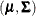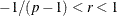### EM Statement

`EM` <options> ;

The expectation-maximization (EM) algorithm is a technique for maximum likelihood estimation in parametric models for incomplete data. The EM statement uses the EM algorithm to compute the MLE for, the means and covariance matrix, of a multivariate normal distribution from the input data set with missing values. Either the means and covariances from complete cases or the means and standard deviations from available cases can be used as the initial estimates for the EM algorithm. You can also specify the correlations for the estimates from available cases.

You can also use the EM statement with the NIMPUTE=0 option in the PROC MI statement to compute the EM estimates without multiple imputation, as shown in Example 57.1.

The following seven options are available with the EM statement (in alphabetical order):

CONVERGE=p
XCONV=p

sets the convergence criterion. The value must be between 0 and 1. The iterations are considered to have converged when the change in the parameter estimates between iteration steps is less than p for each parameter—that is, for each of the means and covariances. For each parameter, the change is a relative change if the parameter is greater than 0.01 in absolute value; otherwise, it is an absolute change. By default, CONVERGE=1E–4.

INITIAL=CC | AC | AC(R=r)

sets the initial estimates for the EM algorithm. The INITIAL=CC option uses the means and covariances from complete cases; the INITIAL=AC option uses the means and standard deviations from available cases, and the correlations are set to zero; and the INITIAL=AC( R= r) option uses the means and standard deviations from available cases with correlation r, whereand p is the number of variables to be analyzed. The default is INITIAL=AC.

ITPRINT

prints the iteration history in the EM algorithm.

MAXITER=number

specifies the maximum number of iterations used in the EM algorithm. The default is MAXITER=200.

OUT=SAS-data-set

creates an output SAS data set that contains results from the EM algorithm. The data set contains all variables in the input data set, with missing values being replaced by the expected values from the EM algorithm. See the section Output Data Sets for a description of this data set.

OUTEM=SAS-data-set

creates an output SAS data set of TYPE=COV that contains the MLE of the parameter vector. These estimates are computed with the EM algorithm. See the section Output Data Sets for a description of this output data set.

OUTITER <( options )> =SAS-data-set

creates an output SAS data set of TYPE=COV that contains parameters for each iteration. The data set includes a variable named `_Iteration_` to identify the iteration number. The parameters in the output data set depend on the options specified. You can specify the MEAN and COV options to output the mean and covariance parameters. When no options are specified, the output data set contains the mean parameters for each iteration. See the section Output Data Sets for a description of this data set.